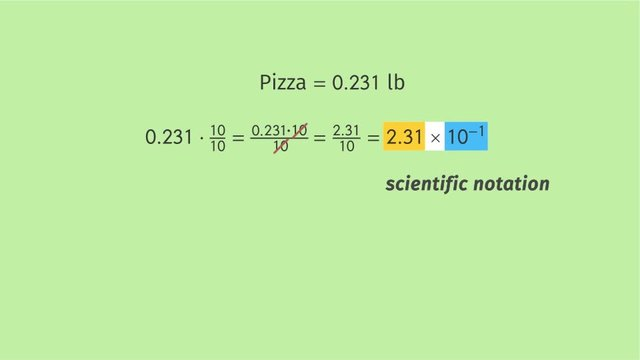# Adding and Subtracting Numbers in Scientific NotationRating

Ø 5.0 / 1 ratings

The authorsChris S.

## Basics on the topicAdding and Subtracting Numbers in Scientific Notation

After this lesson, you will be able to use scientific notation to perform addition and subtraction with extremely large and extremely small numbers.

The lesson begins by teaching you the definition of scientific notation. It leads to converting numbers into scientific notation. It concludes with adding and subtracting numbers in scientific notation.

Learn how to add and subtract using scientific notation by helping the ants and the beetles figure out how to carry the weight of the pizza.

This video includes key concepts, notation, and vocabulary such as scientific notation (a method of writing or displaying numbers in terms of a decimal number between 1 and 10 and multiplied by a power of ten.)

Before watching this video, you should already be familiar with converting from standard to scientific notation, and using order of magnitude to compare numbers.

After watching this video, you will be prepared to learn how to define units by choosing a value in scientific notation and making that a unit.

Common Core Standard(s) in focus: 8.EE.4 A video intended for math students in the 8th grade Recommended for students who are 13 - 14 years old